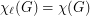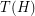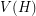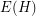Importance: Medium ✭✭
 Author(s): Borodin, Oleg V. Kostochka, Alexandr V. Woodall, Douglas R.
 Subject: Graph Theory » Coloring
 Keywords: list coloring Total coloring total graphs
 Posted by: Jon Noel on: August 29th, 2013
Conjecture   Ifis the total graph of a multigraph, then.

The list chromatic number of a graph, denoted, is defined here. Given a multigraph, the total graphofis a graph on vertex setwhere

\item two elements ofare adjacent inif and only if they are adjacent in; \item two elements ofare adjacent inif and only if they share an endpoint; \item an element ofis adjacent to an element ofinif it is incident with it.

This problem is related to the List (Edge) Colouring Conjecture as well as the Total Colouring Conjecture.

Kostochka and Woodall [KW] conjectured thatfor every graph; this was known as the List Square Colouring Conjecture. It is stronger than the List Total Colouring Conjecture since, given a multigraph, the total graph ofcan be obtained by subdividing each edge ofand taking the square. Moreover, the graph obtained fromby subdividing each edge is bipartite and one part of the bipartition consists of vertices of degree. Thus, the List Total Colouring Conjecture corresponds to this (very) special case of the List Square Colouring Conjecture.

However, the List Square Colouring Conjecture is not true in general. For a family of counterexamples, see the paper of Kim and Park [KP].

## Bibliography

*[BKW] O. V. Borodin, A. V. Kostochka, and D. R. Woodall. List edge and list totalcolourings of multigraphs. J. Combin. Theory Ser. B, 71(2):184–204, 1997.

[KW] A. V. Kostochka and D. R. Woodall. Choosability conjectures and multicircuits. Discrete Math., 240(1-3):123–143, 2001.

[KP] Seog-Jin Kim and Boram Park: Counterexamples to the List Square Coloring Conjecture, submitted.

* indicates original appearance(s) of problem.